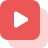Home » About GATE » GATE study materials » Agricultural Engineering » Sequences and Series-Infinite Series

# Sequences and Series-Infinite Series

This article deals with the introduction of sequences and series, types of sequences and series, sequence and series formulas, arithmetic sequences examples, and finite geometric series.

Sequence and Series are some of the topics in arithmetic. An ordered collection of elements in which repetition of any sort is allowed is called a sequence. The sum of all elements is called series. An arithmetic progression is one of the examples of sequence and series.

## Sequence:

A sequence is a list of objects. Just as a set contains elements, the number of elements is called the Length of sequence. Order Is important in sequence, and one element can appear multiple times at different positions in a sequence.  A sequence can also be defined as a function whose domain is countable ordered, such as the natural numbers.

For example (P, A, R, T, Y), (S, U, M, M, E, R ), (1,1,2,3,4,5,6,7) In this example, elements are repeated at different positions, which are the examples of finite sequences. While (2,4,6,8……) is the example of an infinite series.

Infinite sequences are also known as streams, while finite sequences are strings or words. The empty sequence is denoted as { }. These are included in most notions of sequence while excluded depending upon the context.

## Series:

A series is the sum of the elements of a sequence. First and the last terms are defined in the series and finance sequences, while  infinite sequences and series continue without any definite first and last term.

Examples: Infinite sequence: {An } Series of this sequence is a1 , +a2 , +a3 +, ……..

## Types of Sequence and Series:

• ### Arithmetic sequence:

This is obtained by adding or subtracting a definite number to the preceding term.

• ### Geometric Sequence:

This is obtained by multiplying or deciding the preceding number with a definite number.

• ### Harmonic Sequence:

A series of numbers is said to be harmonic if the reciprocals of all the sequence elements form an arithmetic sequence.

• ### Fibonacci Numbers:

An interesting sequence of numbers in which each element is obtained by adding two preceding elements. They start with 0 and 1. They are commonly denoted as Fn.

Example: F0= 0, F1 = 1, F2=1, F3=2, F4=3, F5 =5, F6=8, F7=13, F8=21, F9=34, F10=55, F11=89, F12=144, F13=233, F14=377, F15=610, F16=987, F17=1597, F18=2584, F19=4181.

### Sequence and Series Formula:

#### For Sequence:

Arithmetic progression:  a, a+d, a+2d, a+3d………, a+(n-1) d,…

Geometric progression: A, ar, ar^2, ….., ar^(n-1),…

Common difference or Ratio:

• For Arithmetic Progression,

Successive term-Preceding term

• For Geometric progression,

Successive term/ preceding term

General term:

• For Arithmetic progression,

An  = a + (n-1)d

• For Geometric progression,

An = ar^(n-1)

Nth term from the last term:

• For Arithmetic progression,

An  = 1- (n-1)d

• For Geometric progression,

An  = 1/r^(n-1)

Sum of the first n terms:

• For Arithmetic progression,

Sn  = n/2(2a + (n-1) d)

• For Geometric progression,

Sn =a(1-r^n)/(1-r), if r<1

Sn = a(r^n -1)/(r-1), if r>1

Here, a = first term, d = common difference, r=common ration, n =position of term, l = last term

### Arithmetic Sequence Examples:

There are some arithmetic progression examples:

Example 1: Find whether the sequence is arithmetic or not? Explain why or why not?

Sequence A: -1,-3,-5,-7,…..

Sequence B: -3,0,4,7,……..

Solution: Sequence A is an arithmetic sequence since every pair of consecutive terms has a common difference of -2. That is d = -2.

On the other hand, Sequence B is not arithmetic since there is no common difference between the pairs of consecutive terms of the sequence.

Example 2: Find the next consecutive term in the sequence?

Sequence: 6, -1,4,?

Solution: The sequence has a common difference of 5. To get the next term to add 5 to the previous term. That is you add 5 to 4 to get the required term. 4+5 =9.

Example 3: Find the next two terms in the sequence below?

Sequence: -1/2, -5/6, -7/6,?

Solution: The common difference between the pairs of consecutive terms is -⅓.

We will use this value to get the required terms.

So, -7/6 + (-⅓) = -3/2 and -3/2+(-⅓) = -11/6.

### Finite Geometric Series:

When we sum the terms in a finite geometric sequence, it is called the finite geometric series.

The general formula of this is:

An= a.r ^ (n-1)

Here, n = position of the sequence

An= nth term of the sequence

a= first term

r = constant ratio

Example:  Find the sum of 8 terms of the series? Given a1 =1 and r =2.

Solution: S8 = 1(1-2^8)/(1-2)  =255

### Conclusion:

Sequence and Series are some of the fields in arithmetic. They are used in the areas of finance, physics, and statistics. There are various types of sequences and series such as arithmetic sequence, geometric sequence, harmonic Sequence, and Fibonacci numbers, with each series having its functional fields.